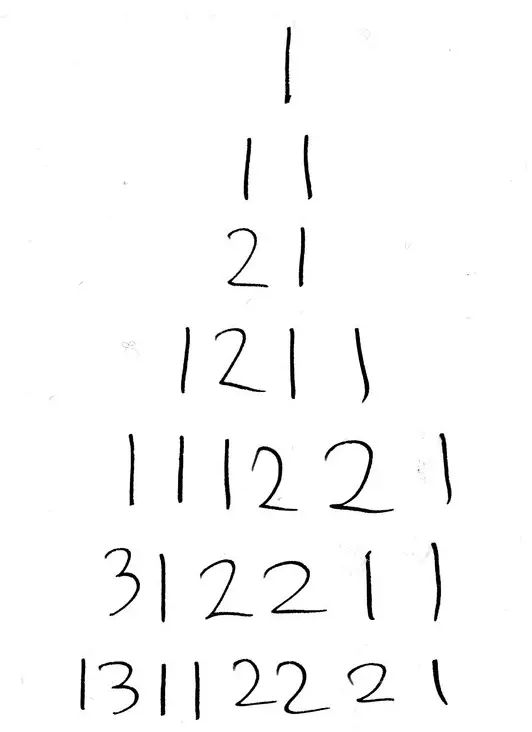# Learning Python with Advent of Code Walkthroughs

Dazbo's Advent of Code solutions, written in Python# Advent of Code 2015 - Day 10

## Problem Intro

This one is quite a simple challenge. We have to read a sequence, and count how many digits there are in any group of the same digit. We say this count aloud, and this forms the next iteration of the sequence. E.g.

• Iteration 1: 1 becomes 11 (1 copy of digit 1).
• Iteration 2: 11 becomes 21 (2 copies of digit 1).
• Iteration 3: 21 becomes 1211 (one 2 followed by one 1).
• Iteration 4: 1211 becomes 111221 (one 1, one 2, and two 1s).

And so on.

## Part 1

How long is our resulting sequence after 40 iterations?

Here’s my function that does the hard work:

``````def look_and_say(data: str) -> str:
""" Perform a single look_and_say iteration

Args:
data (str): The input string, which is a seequence of numbers

Returns:
str: The resulting look-and-say string
"""
digit_counts = []   # store each count as a (count, digit) tuple, e.g. [(3, 1), (2, 2), (1, 1)]
digit_count = 0
prev_digit = None
for digit in data:
if (prev_digit and digit != prev_digit):
# if this digit is different to last digit, store the count of the last digit, and reset the count
digit_counts.append([digit_count, prev_digit])
digit_count = 0

digit_count += 1
prev_digit = digit

digit_counts.append([digit_count, prev_digit])

return "".join(str(count) + str(digit) for count, digit in digit_counts)
``````

It’s pretty simple. It takes a sequence of numeric digits (as a `str`), and outputs the next sequence.

• It iterates through each digit in the sequence.
• If we’ve just read a digit that is different to the previous digit, then:
• We’ve completed our preceding block of 1 or more identical digits.
• Store, in a tuple: `(count, digit)`
• If the current digit is the same as a the previous digit, increment our counter for this block of identical digits.

Finally, when we exit the loop, we use `"".join()` along with a list comprehension, to assemble our new digit sequence from all the `(count, digit)` pairs that we have in our list.

Finally, we want to run this function for the required number of iterations:

``````ITERATIONS = 40

def main():
input_file = os.path.join(SCRIPT_DIR, INPUT_FILE)
with open(input_file, mode="rt") as f:

print(f"Input data: {data}")

for _ in range(ITERATIONS):
data = look_and_say(data)

print(f"After {ITERATIONS} iterations, length of result is {len(data)}")
``````

Easy!

## Part 2

We’re asked to now perform 50 iterations, and then determine the final output length.

We don’t need to change anything except the value of the `ITERATIONS` constant!

My output looks like this:

``````Input data: 1321131112
After 50 iterations, length of result is 6989950
Execution time: 8.8330 seconds
``````

So, it runs in just under 9 seconds. We can probably do better, but this is good enough.